### Digital Logic Circuits–Diode Logic

Diode Logic

In DL (diode logic), all the logic is implemented using diodes and resistors. One basic thing about the diode is that diode needs to be forward biased to conduct. Below is the example of a few DL logic circuits.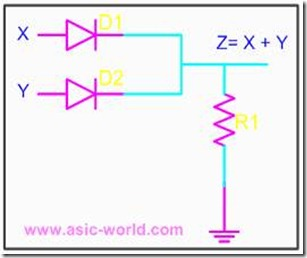When no input is connected or driven, output Z is low, due to resistor R1. When high is applied to X or Y, or both X and Y are driven high, the corresponding diode get forward biased and thus conducts. When any diode conducts, output Z goes high.

Resistor Transistor Logic

In RTL (resistor transistor logic), all the logic are implemented using resistors and transistors. One basic thing about the transistor (NPN), is that HIGH at input causes output to be LOW (i.e. like a inverter). Below is the example of a few RTL logic circuits.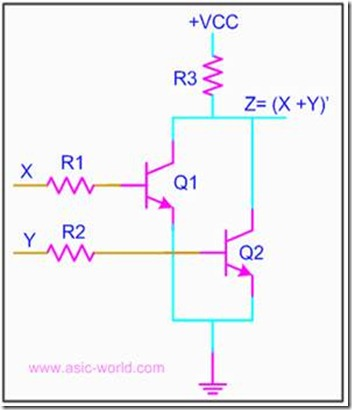A basic circuit of an RTL NOR gate consists of two transistors Q1 and Q2, connected as shown in the figure above. When either input X or Y is driven HIGH, the corresponding transistor goes to saturation and output Z is pulled to LOW.

Diode Transistor Logic

In DTL (Diode transistor logic), all the logic is implemented using diodes and transistors. A basic circuit in the DTL logic family is as shown in the figure below. Each input is associated with one diode. The diodes and the 4.7K resistor form an AND gate. If input X, Y or Z is low, the corresponding diode conducts current, through the 4.7K resistor. Thus there is no current through the diodes connected in series to transistor base. Hence the transistor does not conduct, thus remains in cut-off, and output out is high.

If all the inputs X, Y, Z are driven high, the diodes in series conduct, driving the transistor into saturation. Thus output out is Low.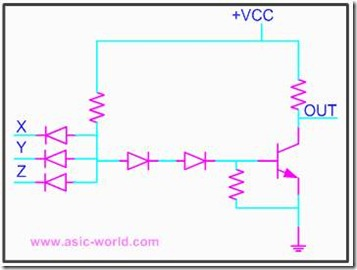Transistor Transistor Logic

In Transistor Transistor logic or just TTL, logic gates are built only around transistors. TTL was developed in 1965. Through the years basic TTL has been improved to meet performance requirements. There are many versions or families of TTL.

· Standard TTL.

· High Speed TTL

· Low Power TTL

· Schhottky TTL

TTL families have three configurations for outputs.

· Totem - Pole output.

· Open Collector Output.

· Tristate Output.

The input stage, which is used with almost all versions of TTL, consists of an input transistor and a phase splitter transistor. Input stage consists of a multi emitter transistor as shown in the figure below. When any input is driven low, the emitter base junction is forward biased and input transistor conducts. This in turn drives the phase splitter transistor into cut-off.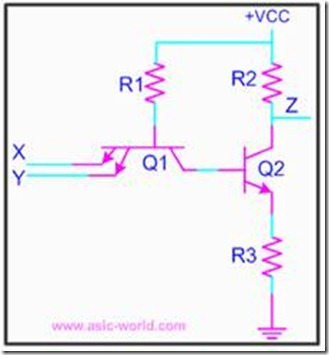Totem - Pole Output

Below is the circuit of a totem-pole NAND gate, which has got three stages.

· Input Stage

· Phase Splitter Stage

· Output Stage

Input stage and Phase splitter stage have already been discussed. Output stage is called Totem-Pole because transistor Q3 sits upon Q4.

Q2 provides complementary voltages for the output transistors Q3 and Q4, which stack one above the other in such a way that while one of these conducts, the other is in cut-off.

Q4 is called pull-down transistor, as it pulls the output voltage down, when it saturates and the other is in cut-off (i.e. Q3 is in cut-off). Q3 is called pull-up transistor, as it pulls the output voltage up, when it saturates and the other is in cut-off (i.e. Q4 is in cut-off).

Diodes in input are protection diodes which conduct when there is large negative voltage at input, shorting it to the ground.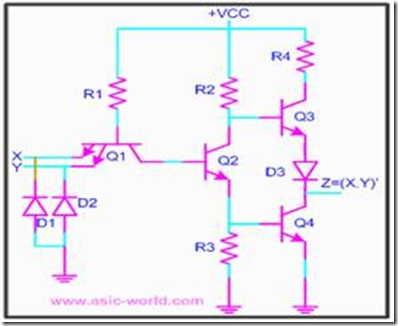Tristate Output.

Normally when we have to implement shared bus systems inside an ASIC or externally to the chip, we have two options: either to use a MUX/DEMUX based system or to use a tri-state base bus system.

In the latter, when logic is not driving its output, it does not drive LOW neither HIGH, which means that logic output is floating. Well, one may ask, why not just use an open collector for shared bus systems? The problem is that open collectors are not so good for implementing wire-ANDs.

The circuit below is a tri-state NAND gate; when Enable En is HIGH, it works like any other NAND gate. But when Enable En is driven LOW, Q1 Conducts, and the diode connecting Q1 emitter and Q2 collector, conducts driving Q3 into cut-off. Since Q2 is not conducting, Q4 is also at cut-off. When both pull-up and pull-down transistors are not conducting, output Z is in high-impedance state.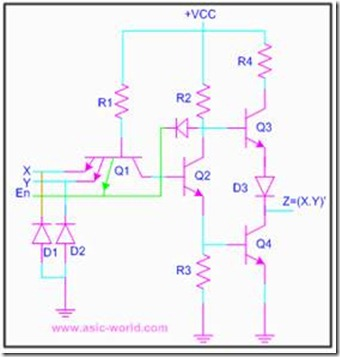Emitter coupled logic

Emitter coupled logic (ECL) is a non saturated logic, which means that transistors are prevented from going into deep saturation, thus eliminating storage delays. Preventing the transistors from going into saturation is accomplished by using logic levels whose values are so close to each other that a transistor is not driven into saturation when its input switches from low to high. In other words, the transistor is switched on, but not completely on. This logic family is faster than TTL.

Voltage level for high is -0.9 Volts and for low is -1.7V; thus biggest problem with ECL is a poor noise margin.

A typical ECL OR gate is shown below. When any input is HIGH (-0.9v), its connected transistor will conduct, and hence will make Q3 off, which in turn will make Q4 output HIGH.

When both inputs are LOW (-1.7v), their connected transistors will not conduct, making Q3 on, which in turn will make Q4 output LOW.

Metal Oxide Semiconductor Logic

MOS or Metal Oxide Semiconductor logic uses nmos and pmos to implement logic gates. One needs to know the operation of FET and MOS transistors to understand the operation of MOS logic circuits.

The basic NMOS inverter is shown below: when input is LOW, NMOS transistor does not conduct, and thus output is HIGH. But when input is HIGH, NMOS transistor conducts and thus output is LOW.

Normally it is difficult to fabricate resistors inside the chips, so the resistor is replaced with an NMOS gate as shown below. This new NMOS transistor acts as resistor.

Complementary Metal Oxide Semiconductor Logic

CMOS or Complementary Metal Oxide Semiconductor logic is built using both NMOS and PMOS. Below is the basic CMOS inverter circuit, which follows these rules:

· NMOS conducts when its input is HIGH.

· PMOS conducts when its input is LOW.

So when input is HIGH, NMOS conducts, and thus output is LOW; when input is LOW PMOS conducts and thus output is HIGH